Test: Problems on AC & DC Analysis

# Test: Problems on AC & DC Analysis

Test Description

## 10 Questions MCQ Test Analog Electronics | Test: Problems on AC & DC Analysis

Test: Problems on AC & DC Analysis for Electrical Engineering (EE) 2023 is part of Analog Electronics preparation. The Test: Problems on AC & DC Analysis questions and answers have been prepared according to the Electrical Engineering (EE) exam syllabus.The Test: Problems on AC & DC Analysis MCQs are made for Electrical Engineering (EE) 2023 Exam. Find important definitions, questions, notes, meanings, examples, exercises, MCQs and online tests for Test: Problems on AC & DC Analysis below.
Solutions of Test: Problems on AC & DC Analysis questions in English are available as part of our Analog Electronics for Electrical Engineering (EE) & Test: Problems on AC & DC Analysis solutions in Hindi for Analog Electronics course. Download more important topics, notes, lectures and mock test series for Electrical Engineering (EE) Exam by signing up for free. Attempt Test: Problems on AC & DC Analysis | 10 questions in 10 minutes | Mock test for Electrical Engineering (EE) preparation | Free important questions MCQ to study Analog Electronics for Electrical Engineering (EE) Exam | Download free PDF with solutions
 1 Crore+ students have signed up on EduRev. Have you?
Test: Problems on AC & DC Analysis - Question 1

### In the circuit, transistor has β =60, VBE=0.7V. Find the collector to emitter voltage drop hoe.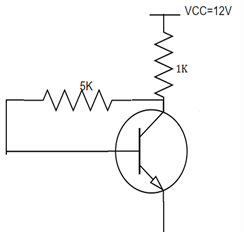Detailed Solution for Test: Problems on AC & DC Analysis - Question 1

We know, IC=(VCC-VBE)/RB
By putting the values, we have IC=5.9mA. IE=IC/α. So, IE=5.99mA.
hoe= VCC-RC(IC+IB). We have hoe=6V.

Test: Problems on AC & DC Analysis - Question 2

### For the circuit shown, find the quiescent point.​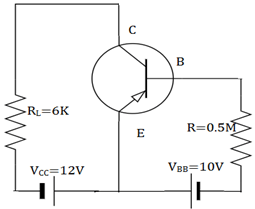Detailed Solution for Test: Problems on AC & DC Analysis - Question 2

We know, hoe=12V
(IC)SAT =VCC/RL=12/6K=2mA. IB=10V/0.5M=20µA. IC= βIB=1mA. I
hoe=VCC-ICRL=12-1*6=6V. So, quiescent point is (6V, 1mA).

Test: Problems on AC & DC Analysis - Question 3

### For the circuit shown, find the quiescent point.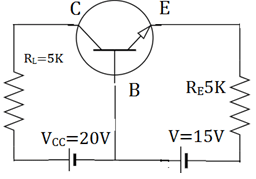Detailed Solution for Test: Problems on AC & DC Analysis - Question 3

We know, IE=VEE/RE=10/5kΩ=2mA
IC=α IE =IE =2mA
VCB=VCC-ICRL=20-10=10V. So, quiescent point is (10V, 2mA).

Test: Problems on AC & DC Analysis - Question 4

In the circuit shown below, β =100 and VBE=0.7V. The Zener diode has a breakdown voltage of 6V. Find the operating point.​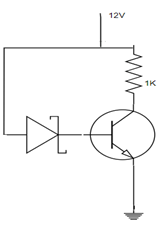Detailed Solution for Test: Problems on AC & DC Analysis - Question 4

We know, by KVL -12+(IC+IB)1K+6+VBE=0
We have IE=5.3. IC= αIE=5.24mA. From another loop, -12+IEIK+VBE=0
We have, hoe=12-5.3m*1000=6.7V. Hence the Q point is (6.7V, 5.3mA).

Test: Problems on AC & DC Analysis - Question 5

10. When the β value is large for a given transistor, the IC and hoe values are given by_________

Detailed Solution for Test: Problems on AC & DC Analysis - Question 5

The base current IB is zero when β value is large. So, the hoe changes to VCC-RCIC. The collector current IC is changed to (VCC-VBE)/RB from β(VCC-VBE)/(1+ β)RE+ RB.

Test: Problems on AC & DC Analysis - Question 6

For the circuit shown, find the quiescent point.​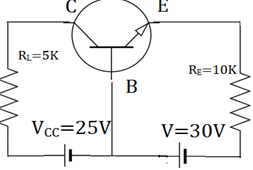Detailed Solution for Test: Problems on AC & DC Analysis - Question 6

We know, IE=VEE/RE=30/10kΩ=3mA
IC=α IE =IE =3mA
VCB=VCC-ICRL=25-15=10V. So, quiescent point is (10V, 3mA).

Test: Problems on AC & DC Analysis - Question 7

The PNP transistor when used for switching the power, then it is called_________

Detailed Solution for Test: Problems on AC & DC Analysis - Question 7

Sometimes DC current gain of a bipolar transistor is too low to directly switch the load current or voltage, so multiple switching transistors is used. The load is connected to ground and the transistor switches the power to it.

Test: Problems on AC & DC Analysis - Question 8

In which of the regions, the small capacitors are open circuited?

Detailed Solution for Test: Problems on AC & DC Analysis - Question 8

In low frequency region, the small capacitors are open circuited and large capacitors are in active state. In high frequency region, the large capacitors are short circuited and small capacitors are active.

Test: Problems on AC & DC Analysis - Question 9

In mid frequency region, the large capacitors are short and small capacitors are open circuited. What happens to the RC coupled circuit?

Detailed Solution for Test: Problems on AC & DC Analysis - Question 9

In the mid frequency region, the whole circuit is resistive because the large capacitors are short and small capacitors are open circuited. The gain is constant in this region. So, the circuit is frequency blind as the gain is constant in this region.

Test: Problems on AC & DC Analysis - Question 10

In low frequency region, the gain_________

Detailed Solution for Test: Problems on AC & DC Analysis - Question 10

At very low frequency, the gain decreases due to the coupling capacitor. When the frequency is decreased, the reactance of the circuit increases and the drop across the coupling capacitor increases. The gain is therefore decreased as the output decreased.

## Analog Electronics

11 videos|57 docs|108 tests
 Use Code STAYHOME200 and get INR 200 additional OFF Use Coupon Code
Information about Test: Problems on AC & DC Analysis Page
In this test you can find the Exam questions for Test: Problems on AC & DC Analysis solved & explained in the simplest way possible. Besides giving Questions and answers for Test: Problems on AC & DC Analysis, EduRev gives you an ample number of Online tests for practice

## Analog Electronics

11 videos|57 docs|108 tests